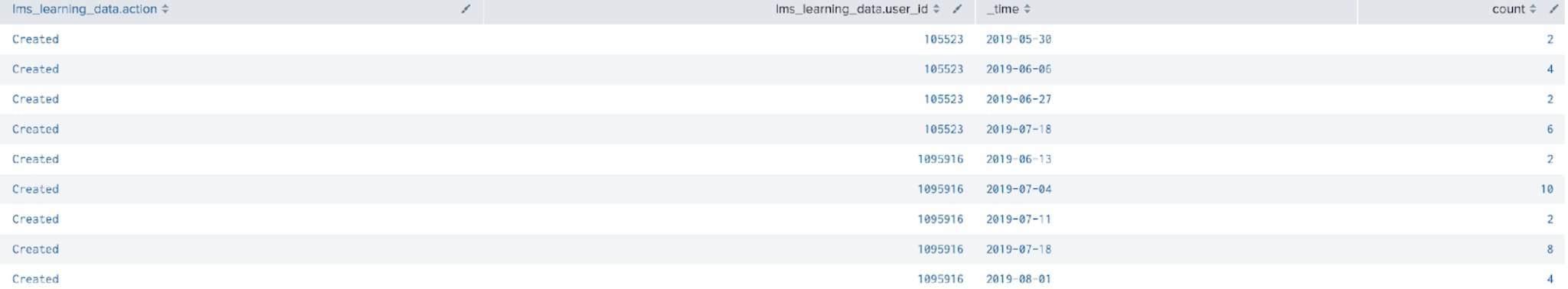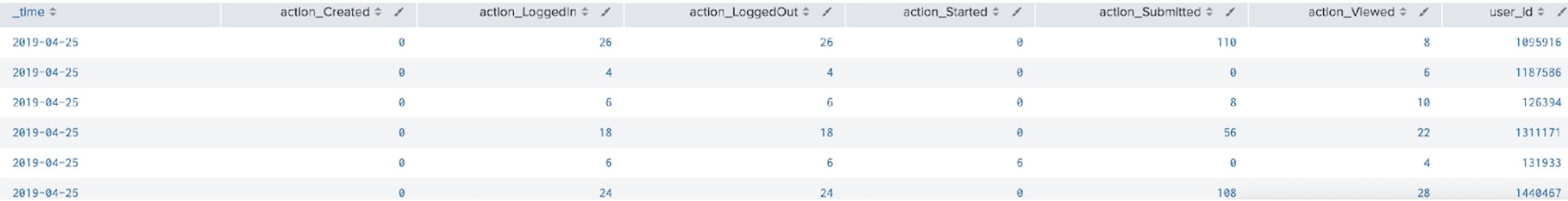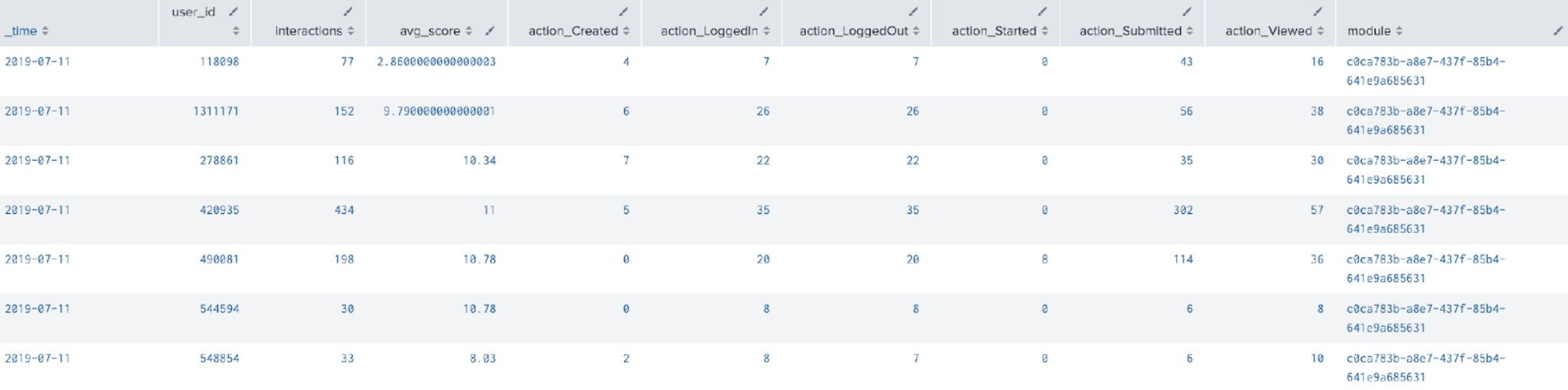PLATFORM

# Smart Education Insights App for Splunk – Part II

Hopefully, you will have had a chance to read through the first half of this blog series, which described how to use the Smart Education Insights App for Splunk. In this blog, we will describe how to apply feature engineering to some example datasets taken from higher education organisations, which can then be used to generate predictive models in the Smart Education Insights app.

Feature engineering is really just a fancy term for transforming a dataset, though usually it is applied to transform the dataset into something that better represents some of the underlying behaviour of the data. In Splunk terms, you can think of using a transforming command like stats or even a simple eval function as ways to engineer features in data.

Here we will describe some example searches that we have used to extract features from Virtual Learning Environment (VLE) data. Although we recognise that not every university will have data that looks exactly like what we will be using here, hopefully, this blog will offer some helpful hints and techniques for evaluating similar features in different datasets.

## Preparing your data for machine learning

We are going to look at a couple of examples in this blog:

We’ll begin with the OULAD dataset and explain the search that we used to generate the dataset that comes with the app.

There are a set of sources that come in the OULAD dataset, and here we are using the following files:

• studentVle.csv, which contains information about interactions with the VLE for each course and each student over time
• studentInfo.csv, which contains contextual information about each student, such as previous qualifications and course results
• assessment.csv, which contains assessment results over time for each course and each student

Note that for the VLE and assessment data we have converted the times in the original data into something that Splunk can interpret correctly before ingesting and that both of these data sources have been ingested into an index called oulad. The studentInfo.csv file has been imported into Splunk as a lookup file for this particular search.

To begin with, we are simply going to search the data for one module (AAA) and create a couple of new fields: a weighted score to represent the exact contribution to the students' final result, and an ID field that comprises the student ID, course ID and presentation ID. The search for this is below:

```index=oulad code_module=AAA
| eval weighted_score=score*(weight/100), student_code=id_student."_".code_module."_".code_presentation
```

Next, we are going to calculate some statistics from these search results for every week:

```...
| bin _time span=1w
| stats sum(sum_click) as sum_clicks sum(weighted_score) as week_score avg(score) as average_score by student_code _time
| streamstats sum(week_score) as cumulative_score last(average_score) as last_average count by student_code
| eventstats max(count) as course_length
| eval average_score=if(average_score>0,average_score,if(last_average>0,last_average,0)), cumulative_score=if(cumulative_score>0,cumulative_score,0), module_perc_complete=count/course_length
```

The stats command gives us the total number of interactions with the VLE (sum_clicks), the in-week contribution to the students' final score (week score), and the average score for each student on the module too (average_score). Streamstats is then being used to calculate the cumulative score for each student and also fill in missing values with the previous average score if there weren’t any assessment in-week. The eventstats command then collects how long the course runs for, and this is then used in an eval statement to calculate how far through the module the current week is. The eval command also replaces missing average score and cumulative scores with zero, which ensures the first few weeks of data aren’t filled with null values for these fields.

The next code snippet below enriches our results with some context about the students – critically this also adds in the result each student achieved on the course. This data is then pushed out to a lookup file, which is the same file that can be found in the app itself.

```…
| join student_code [| inputlookup student_info.csv | eval student_code=id_student."_".code_module."_".code_presentation | table student_code age_band highest_education imd_band studied_credits final_result]
| table _time student_code sum_clicks average_score cumulative_score module_perc_complete studied_credits age_band highest_education imd_band final_result
```

It is the results of this data that is used to generate the predictive models in the app if you choose to use this example dataset to test the functionality out.

### Student Success Toolkit

We’re now going to look at how the data models in the SSTK can be used to generate features that could be used in the app for generating a predictive model.

There are two main data models in the SSTK that we are using here:

• The lms_learning_data data model, which contains details of how students are interacting with the VLE over time
• The lms_scoring data model, which contains details of student grades over time

Provided you are using the SSTK these data models should be available for you to use.

To begin with we are going to extract data for one course from the learning data data model:

```| datamodel lms_learning_data search
| search
"lms_learning_data.course_id"="c0ca783b-a8e7-437f-85b4-641e9a685631"
```

We’re using the course ID here, but you could use the course name as a filter as well.

From this data we are going to calculate for each week how many interactions each student is having with the VLE broken down by each action they can perform:

```…
| bin _time span=1w
| stats count by lms_learning_data.action lms_learning_data.user_id _time
```We then transform this data into a more tabular format using xyseries, but in order to preserve both the time and student ID data we are first going to concatenate those fields, before splitting them out again after we have used xyseries:

```…
| eval x='_time'."_".'lms_learning_data.user_id', lms_learning_data.action="action_".'lms_learning_data.action'
| xyseries x lms_learning_data.action count
| fillnull value=0
| makemv delim="_" x
| eval _time=mvindex(x,0), user_id=mvindex(x,1)
| fields - x
```Next up we calculate the total number of interactions (regardless of the action) with the VLE:

```…
| eval interactions=0
| foreach action_*
[| eval interactions=interactions+<<FIELD>>]
```

Finally, we are going to enrich this data with some average scores for each student by using the lms_scoring data model. We will use the join command here, and merge the average score for each student over each week with the results we already have. Note that we are also filtering the lms_scoring data model to only return data from the course with the same ID as above:

```…
| join _time user_id
[| datamodel lms_scoring search
| search "lms_scoring.course_id"="c0ca783b-a8e7-437f-85b4-641e9a685631"
| bin _time span=1w
| stats avg(lms_scoring.score) as avg_score by _time lms_scoring.user_id
| rename lms_scoring.user_id as user_id]
| table _time user_id interactions avg_score action_*
| eval module="c0ca783b-a8e7-437f-85b4-641e9a685631"
```The one key bit of information we are missing here is the result that each student obtained on the course. We’d recommend that you add this data in using either a lookup – as we did in the example for the OULAD data above) or a join, depending on the size of the results data and how it has been sourced.

## Summary

Hopefully, this blog has provided some useful techniques for preparing your data for use in the Smart Education Insights App for Splunk. I’d encourage you to download the app and try out using the content yourself with some of these searches!Posted by

#### Greg Ainslie-Malik

Greg is a Machine Learning Architect at Splunk where he helps customers deliver advanced analytics and uncover new ways of insight from their data. Prior to working at Splunk he spent a number of years with Deloitte and before that BAE Systems Detica working as a data scientist. Before getting a proper job he spent way too long at university collecting degrees in maths including a PhD on “Mathematical Analysis of PWM Processes”. When he is not at work he is usually herding his three young lads around while thinking that work is significantly more relaxing than being at home…

TAGS

Show All Tags
Show Less Tags